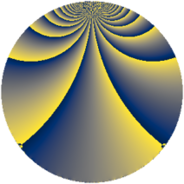# Properties

 Label 288.3.uLevel $288$ Weight $3$ Character orbit 288.u Rep. character $\chi_{288}(19,\cdot)$ Character field $\Q(\zeta_{8})$ Dimension $156$ Newform subspaces $3$ Sturm bound $144$ Trace bound $14$

# Related objects

## Defining parameters

 Level: $$N$$ $$=$$ $$288 = 2^{5} \cdot 3^{2}$$ Weight: $$k$$ $$=$$ $$3$$ Character orbit: $$[\chi]$$ $$=$$ 288.u (of order $$8$$ and degree $$4$$) Character conductor: $$\operatorname{cond}(\chi)$$ $$=$$ $$32$$ Character field: $$\Q(\zeta_{8})$$ Newform subspaces: $$3$$ Sturm bound: $$144$$ Trace bound: $$14$$ Distinguishing $$T_p$$: $$5$$

## Dimensions

The following table gives the dimensions of various subspaces of $$M_{3}(288, [\chi])$$.

Total New Old
Modular forms 400 164 236
Cusp forms 368 156 212
Eisenstein series 32 8 24

## Trace form

 $$156q + 4q^{2} - 4q^{4} + 4q^{5} - 4q^{7} + 4q^{8} + O(q^{10})$$ $$156q + 4q^{2} - 4q^{4} + 4q^{5} - 4q^{7} + 4q^{8} + 36q^{10} + 4q^{11} - 4q^{13} - 12q^{14} - 4q^{19} + 84q^{20} - 80q^{22} - 60q^{23} - 4q^{25} + 104q^{26} + 56q^{28} + 4q^{29} - 16q^{32} - 48q^{34} + 100q^{35} - 4q^{37} + 116q^{38} + 40q^{40} + 4q^{41} + 92q^{43} + 84q^{44} + 28q^{46} + 8q^{47} + 4q^{50} - 428q^{52} - 156q^{53} - 260q^{55} - 160q^{56} - 176q^{58} + 132q^{59} + 60q^{61} - 168q^{62} - 136q^{64} + 8q^{65} - 36q^{67} - 488q^{68} + 8q^{70} - 252q^{71} - 4q^{73} - 524q^{74} - 308q^{76} + 228q^{77} + 504q^{79} - 640q^{80} - 404q^{82} + 484q^{83} + 96q^{85} - 240q^{86} + 112q^{88} + 4q^{89} + 188q^{91} + 168q^{92} + 760q^{94} - 8q^{97} + 56q^{98} + O(q^{100})$$

## Decomposition of $$S_{3}^{\mathrm{new}}(288, [\chi])$$ into newform subspaces

Label Dim. $$A$$ Field CM Traces $q$-expansion
$$a_2$$ $$a_3$$ $$a_5$$ $$a_7$$
288.3.u.a $$28$$ $$7.847$$ None $$4$$ $$0$$ $$4$$ $$-4$$
288.3.u.b $$64$$ $$7.847$$ None $$0$$ $$0$$ $$0$$ $$0$$
288.3.u.c $$64$$ $$7.847$$ None $$0$$ $$0$$ $$0$$ $$0$$

## Decomposition of $$S_{3}^{\mathrm{old}}(288, [\chi])$$ into lower level spaces

$$S_{3}^{\mathrm{old}}(288, [\chi]) \cong$$ $$S_{3}^{\mathrm{new}}(32, [\chi])$$$$^{\oplus 3}$$$$\oplus$$$$S_{3}^{\mathrm{new}}(96, [\chi])$$$$^{\oplus 2}$$